FORM 2 PHYSICS

ONLINE TEST SERIES

HOOKES LAW

1. The diagram shows how the length of a spring changes when a load of 10 N is hung on it
2.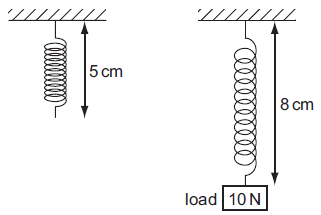3. The 10 N load is replaced by a 20 N load. What is the new length of the spring?

1. A spring balance is calibrated to give readings in newtons. The graph shows how the extension of the spring varies with the load.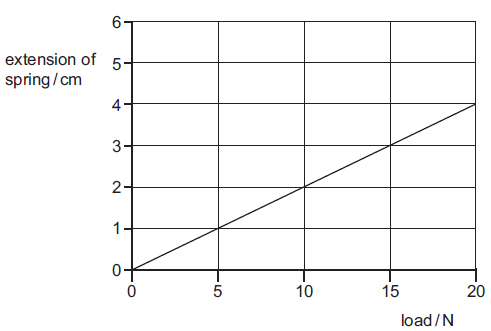A load causes the spring of the balance to extend by 3 cm.

1. A student adds weights to an elastic cord. He measures the length of the cord for each weight. He then plots a graph from the results, as shown below..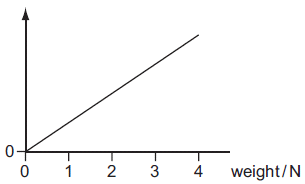Which length has he plotted on the vertical axis?

4. An extension-load graph for a wire is shown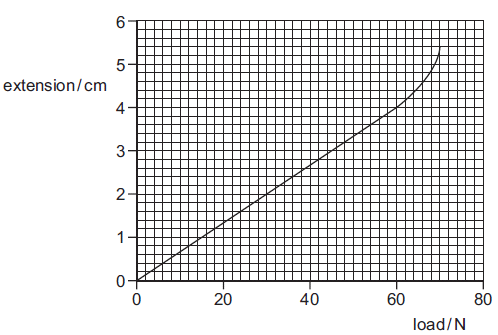What is the load at the limit of proportionality for the wire?

1. A 100 g mass is suspended from a spring next to a vertical metre rule. The top of the spring is level with the 0.0 cm mark. The bottom of the spring is level with the 27.2 cm mark.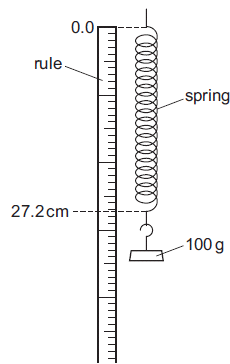The 100 g mass is replaced with a 600 g mass. The length of the spring is now 89.7 cm. The spring has not reached the limit of proportionality.The 600 g mass is replaced with a 200 g mass. What is the length of the spring?

 6 Which formula correctly states the relationship between force and extension?

 7 If a material has a spring constant of 10N/m and is extended by 1m, what force must have been applied to the object?

 8 What is the size of the extension of an object which has a spring constant of 30N/m when a force of 15N is applied?

 9 A slinky is stretched from being a length of 15cm to a length of 2m. One end of the slinky is affixed to a point whilst a child pulls on the other end with a force of 10N. What is the spring constant of the slinky?

 10 An spring is stretched to a point where, when released it does not return to its original length. What is this point called?

Question 1 of 10